# Electronics and Communication Engineering - Electromagnetic Field Theory

31.

Calculate the wave impedance for TM mode in rectangular waveguide for dominant mode at 3 GHz having

 A. (120p) Ω B. 377 Ω C. 226 Ω D. 629 Ω

Explanation: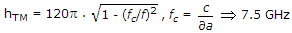η = 226 Ω.

32.

The divergence of the electric field intensity at any point equals

 A. 1/4 pε0 times the volume charge density B. l/ε0 times the volume charge density at that point C. ε0 times the volume charge density at that point D. the volume charge density at that point

Explanation: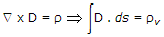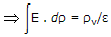33.

For a 400 kHz transmission line having L = 0.5 mH/km, C = 0.08 mF and negligible R and G, the value of propagation constant P will be

Explanation:

γ = a + jβ, a = 0 β = ωLC.

34.

The Depth of penetration of EM wave in medium having conductivity σ at a frequency of 1 MHz is 25 cm. The depth of penetration at a frequency of 4 MHz will be

 A. 6.25 cm B. 12.50 cm C. 50 cm D. 100 cm

Explanation:

Skin depth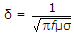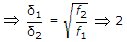δ2 = δ1/2 .

35.

What is the characteristic impedance at 10 MHz?

 A. 547.7 Ω B. 54.7 Ω C. 5.47 Ω D. .547 Ω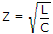, not depend upon frequency.